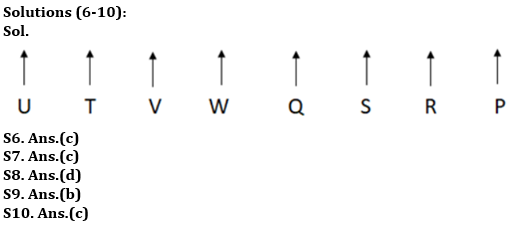Latest Banking jobs   »

# Reasoning Quiz For RBI Grade B Phase 1 2023-12th May

Directions (1-5): Study the numbers sequence carefully and answer the questions given below.

759 594 396 444 372 423

Q1. What is the resultant if the first digit of the second-highest number is divided by the first digit of the second-lowest number?
(a) 2.1
(b) 1.8
(c) 2.3
(d) 1.6
(e) None

Q2. If one is subtracted from all even digits in the numbers, then in how many numbers, a digit appears more than once within the number?
(a) Two
(b) One
(c) Four
(d) Three
(e) None of these

Q3. If all numbers are arranged in ascending order from the left to the right, then what is the difference between the numbers which are third from the left end and second from the right end?
(a) 190
(b) 171
(c) 221
(d) 118
(e) None of these

Q4. If in each number, 1 is added to the first digit and then the first and the third digits are interchanged within the number then which of the following will be the largest number?
(a) 423
(b) 594
(c) 759
(d) 444
(e) None of these

Q5. If the positions of the first and the second digits are interchanged in each number, then which of the following will be the lowest number?
(a) 444
(b) 396
(c) 372
(d) 423
(e) None of these

Directions (6-10): Study the following information carefully and answer the questions accordingly.
Eight people P, Q, R, S, T, U, V, and W are sitting in a line facing the north direction but not necessarily in the same order. R is not sitting at an end. W sits second to the right of T. Three people are sitting between Q and U. S sits third to the right of V. Two people are sitting between Q and T who is not sitting at an end. U is sitting at an end.

Q6. Who sits second to the left of S?
(a) U
(b) Q
(c) W
(d) P
(e) None of these

Q7. How many people are sitting between U and Q?
(a) Two
(b) Four
(c) Three
(d) One
(e) None of these

Q8. Who sits fourth to the right of T?
(a) P
(b) R
(c) U
(d) S
(e) None of these

Q9. How many of them sit to the left of R?
(a) Four
(b) Six
(c) Five
(d) One
(e) None of these

Q10. Which among the following does not belong to the group of other four?
(a) P, S
(b) V, Q
(c) R, P
(d) U, V
(e) S, W

Solutions

Solutions (1-5):
S1. Ans.(d)
Sol. Given Series = 759 594 396 444 372 423
second-highest number- 594
second-lowest number- 396
Resultant = 5 ÷ 3 = 1.6

S2. Ans.(a)
Sol. Given Series = 759 594 396 444 372 423
After subtraction = 759 593 395 333 371 313
Therefore, option (a) is the correct answer.

S3. Ans.(b)
Sol. Given Series = 759 594 396 444 372 423
After rearrangement = 372 396 423 444 594 759
Required difference = 594 – 423 = 171
Therefore, option (b) is the correct answer.

S4. Ans.(c)
Sol. Given Series = 759 594 396 444 372 423
After adding 1 = 859 694 496 544 472 523
After interchange = 958 496 694 445 274 325
Therefore, option (c) is the answer.

S5. Ans.(d)
Sol. Given Series = 759 594 396 444 372 423
After interchange = 579 954 936 444 732 243
Therefore, option (d) is the correct answer.## FAQs

### What is the selection process of the Bank Clerk?

There are 4 sections in the RBI Grade B Phase 1 Exam i.e. English Language, General Awareness, Quantitative Aptitude & Reasoning.

#### Congratulations!Union Budget 2023-24: Free PDF